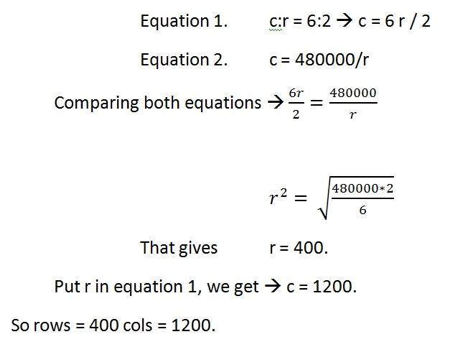# Images-Informational Sheet

Images: The Rundown of the Basics

Created: April 2019

Modified:

This paper is to just give you some basic terms and ideas about Images.

An image is a visual representation of something, you probably would recognize this most commonly as pictures. Images on a screen are typically composed or made up of pixels. Pixels are the smallest points that make up the hue and brightness of an image. When you use a camera to take a picture your camera takes the photo at a fixed resolution (size and definition), meaning there are only so many pixels contained within an image. A pixel can be seen when you Zoom into a photo to the point that the image you see on the screen becomes blocky which is known as Aliasing.

There are two types of images, pixel based images that are called Raster images and Vector based images, which are based on mathematics. Pixel based images contain a finite number of pixels. Vector images are much more flexible and computational and therefore can be infinite, meaning that they will always look crisp no matter how you zoom into them.

Raster and Vector images each have several unique file formats that they can be saved as from your program or application you use to construct and/or edit them. Raster images use JPG, PNG, GIF, TIFF and many more (see below for clarification). Vector images are typically saved in EPS, Adobe PDF*, AI, SVG and others.

Common Types of File Formats

• JPEG (or JPG) - Joint Photographic Experts Group. Can be compressed to save file space.
• PNG - Portable Network Graphics. PNGs are amazing for interactive documents such as web pages and also show transparency.
• GIF - Graphics Interchange Format. GIFs are most common in their animated form...
• TIFF - Tagged Image File. A TIF is a large raster file that doesn't lose quality. Can be very large files.
• .EPS - Encapsulated PostScript. Self contained files for printing typically.
• .AI - Adobe Illustrator file format is a proprietary format that because of its popularity is well used.
• .SVG - Scalable Vector Graphics. Open standard developed by the World Wide Web Consortium (W3C) since 1999. SVG images are based in XML and are images that can be interactive and animated.

*A PDF is generally a vector file. However, depending how a PDF is originally created, it can be either a vector or a raster file.  Whether you opt to flatten the layers of your file or choose to retain each one will determine the image type.

https://modassicmarketing.com/understanding-image-file-typesThe math in Images

Info derived from https://www.tutorialspoint.com/dip/index.htm

Bpp or bits per pixel denotes the number of bits per pixel. The number of different colors in an image is depends on the depth of color or bits per pixel.

Bits in mathematics:

Its just like playing with binary bits.

How many numbers can be represented by one bit.

0

1

How many two bits combinations can be made.

00

01

10

11

If we devise a formula for the calculation of total number of combinations that can be made from bit, it would be like this.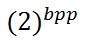Where bpp denotes bits per pixel. Put 1 in the formula you get 2, put 2 in the formula, you get 4. It grows exponentially.

Number of different colors:

Now as we said it in the beginning, that the number of different colors depend on the number of bits per pixel.

The table for some of the bits and their color is given below.

 Bits per pixel Number of colors 1 bpp 2 colors 2 bpp 4 colors 3 bpp 8 colors 4 bpp 16 colors 5 bpp 32 colors 6 bpp 64 colors 7 bpp 128 colors 8 bpp 256 colors 10 bpp 1024 colors 16 bpp 65536 colors 24 bpp 16777216 colors (16.7 million colors) 32 bpp 4294967296 colors (4294 million colors)

This table shows different bits per pixel and the amount of color they contain.

You can easily notice the pattern of the exponentional growth. The famous gray scale image is of 8 bpp , means it has 256 different colors in it or 256 shades.Color images are usually of the 24 bpp format, or 16 bpp.

We will see more about other color formats and image types in the tutorial of image types.

Color values:

We have previously seen in the tutorial of concept of pixel, that 0 pixel value denotes black color.

Black color:

Remember, 0 pixel value always denotes black color. But there is no fixed value that denotes white color.

White color:

The value that denotes white color can be calculated as :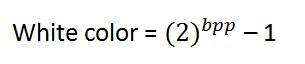In case of 1 bpp, 0 denotes black, and 1 denotes white.

In case 8 bpp, 0 denotes black, and 255 denotes white.

Gray color:

When you calculate the black and white color value, then you can calculate the pixel value of gray color.

Gray color is actually the mid point of black and white. That said,

In case of 8bpp, the pixel value that denotes gray color is 127 or 128bpp (if you count from 1, not from 0).

Image storage requirements

After the discussion of bits per pixel, now we have every thing that we need to calculate a size of an image.

Image size

The size of an image depends upon three things.

• Number of rows
• Number of columns
• Number of bits per pixel

The formula for calculating the size is given below.

Size of an image = rows * cols * bpp

It means that if you have an image, lets say this one: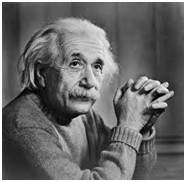Assuming it has 1024 rows and it has 1024 columns. And since it is a gray scale image, it has 256 different shades of gray or it has bits per pixel. Then putting these values in the formula, we get

Size of an image = rows * cols * bpp

= 1024 * 1024 * 8

= 8388608 bits.

But since its not a standard answer that we recognize, so will convert it into our format.

Converting it into bytes = 8388608 / 8 = 1048576 bytes.

Converting into kilo bytes = 1048576 / 1024 = 1024kb.

Converting into Mega bytes = 1024 / 1024 = 1 Mb.

Thats how an image size is calculated and it is stored. Now in the formula, if you are given the size of image and the bits per pixel, you can also calculate the rows and columns of the image, provided the image is square(same rows and same column).

Pixel

We have already defined a pixel in our tutorial of concept of pixel, in which we define a pixel as the smallest element of an image. We also defined that a pixel can store a value proportional to the light intensity at that particular location.

Now since we have defined a pixel, we are going to define what is resolution.

Resolution

The resolution can be defined in many ways. Such as pixel resolution, spatial resolution, temporal resolution, spectral resolution. Out of which we are going to discuss pixel resolution.

You have probably seen that in your own computer settings, you have monitor resolution of 800 x 600, 640 x 480 e.t.c

In pixel resolution, the term resolution refers to the total number of count of pixels in an digital image. For example. If an image has M rows and N columns, then its resolution can be defined as M X N.

If we define resolution as the total number of pixels, then pixel resolution can be defined with set of two numbers. The first number the width of the picture, or the pixels across columns, and the second number is height of the picture, or the pixels across its width.

We can say that the higher is the pixel resolution, the higher is the quality of the image.

We can define pixel resolution of an image as 4500 X 5500.

Megapixels

We can calculate mega pixels of a camera using pixel resolution.

Column pixels (width ) X row pixels ( height ) / 1 Million.

The size of an image can be defined by its pixel resolution.

Size = pixel resolution X bpp ( bits per pixel )

Calculating the mega pixels of the camera

Lets say we have an image of dimension: 2500 X 3192.

Its pixel resolution = 2500 * 3192 = 7982350 bytes.

Dividing it by 1 million = 7.9 = 8 mega pixel (approximately).

Aspect ratio

Another important concept with the pixel resolution is aspect ratio.

Aspect ratio is the ratio between width of an image and the height of an image. It is commonly explained as two numbers separated by a colon (8:9). This ratio differs in different images, and in different screens. The common aspect ratios are:

1.33:1, 1.37:1, 1.43:1, 1.50:1, 1.56:1, 1.66:1, 1.75:1, 1.78:1, 1.85:1, 2.00:1, e.t.c

Aspect ratio maintains a balance between the appearance of an image on the screen, means it maintains a ratio between horizontal and vertical pixels. It does not let the image to get distorted when aspect ratio is increased.

For example

This is a sample image, which has 100 rows and 100 columns. If we wish to make is smaller, and the condition is that the quality remains the same or in other way the image does not get distorted, here how it happens.

Original image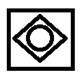Changing the rows and columns by maintain the aspect ratio in MS Paint.

Result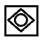Smaller image, but with same balance.

You have probably seen aspect ratios in the video players, where you can adjust the video according to your screen resolution.

Finding the dimensions of the image from aspect ratio:

Aspect ratio tells us many things. With the aspect ratio, you can calculate the dimensions of the image along with the size of the image.

For example

If you are given an image with aspect ratio of 6:2 of an image of pixel resolution of 480000 pixels given the image is an gray scale image.

And you are asked to calculate two things.

• Resolve pixel resolution to calculate the dimensions of image
• Calculate the size of the image

Solution:

Given:

Aspect ratio: c:r = 6:2

Pixel resolution: c * r = 480000

Bits per pixel: grayscale image = 8bpp

Find:

Number of rows = ?

Number of cols = ?

Solving first part: